## Counting in Year 1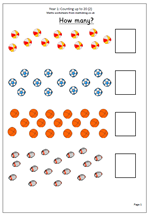It is often assumed that children in year 1 are capable at counting, but this is frequently not the case and they need plenty of practice reciting the number names and counting on and back.

Of course number rhymes such as ‘One, two, three, four, five, Once I caught a fish alive’ will help with this.

There are plenty of occasions when counting can be done in a practical situation, such as counting out knives and forks, counting objects, recounting them if they have been rearranged etc.

This worksheet looks at counting sets of objects with numbers up to 20. They balls etc have been arranged so that they do not line up neatly, which makes it harder for them to be counted.

Count up to 20 (2)

## Resource of the Week: Find tenths and hundredths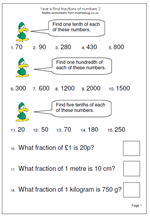This week we take a look at a worksheet on the relationship between fractions and division. It is important that children understand that finding one half of a number is equivalent to dividing by 2, that finding one tenth of a number is the same as dividing the number by 10 and to find one hundredth is equivalent to dividing by 100. Plenty of practice is needed with this before children go on to find, for example, two tenths, or three hundredths of numbers.

Most of these questions should be calculated mentally, although rough jottings may help. The second set of questions could cause a few problems. For example: ‘What fraction of a £1 is 20 ?’ requires a couple of steps:

Firstly convert the pound to pence £1 = 100p

Secondly write the fraction as 20 out of 100 or 20/100

Thirdly simplify to 2/10 and then 1/5.

Find fractions of numbers (p2)

## Square numbers and square roots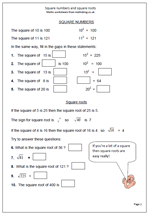A square number is a whole number multiplied by itself for example 3 x 3 = 9. This makes 9 a square number. By Year 6 most children will be familiar with square numbers and hopefully will know the first ten or so square numbers off be heart as they all appear in the times tables.

The square root of a number is a value, or number, that can be multiplied by itself to give the original number.

For example: the square root of 16 is 4 because 4 x 4 = 16

The square root of a number is usually written using the ‘tick’ square root sign. Each whole number is the square root of another number:

5 is the square root of 25

6 is the square root of 36 etc

This page takes a quick look at square numbers and square roots and can be found in our Year 6, Knowing Number facts section.

Square numbers and square roots

## Resource of the week: year 5 square numbers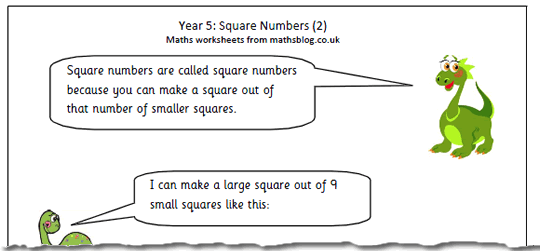This is just one of a short series of square number maths worksheets for Year 5.  It is an interesting and worthwhile exercise asking children to see if they can make a square out of 10 or 12 smaller squares (not overlapping etc) using plastic or card squares. Rectangles are possible, but not squares.

They can then be asked to find which numbers can be made into a square. This can be done either with smaller squares or as dots in an array.

There are several ways that questions involving square numbers can be phrased, including:

What is 4 squared?

What is the square of 4?

What number multiplied by itself makes 16?

Square numbers 2

## Year 3 maths worksheet: adding 3 numbers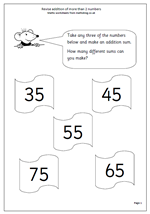Here we have another page of addition which looks at adding three numbers. Each number is a multiple of 5, but there is an extra twist in that children need to work out how many different additions can be made from the numbers available.

A systematic approach is needed, perhaps starting with the largest three numbers;

i.e. 75 + 65 + 55

then moving on to the next largest;

i.e. 75 + 65 + 45

and so on.

An important part of this is to understand that addition can be done in any order so 75 + 65 + 55 is the same as 55 + 65 + 75.

## Divide 3-digit numbers by 7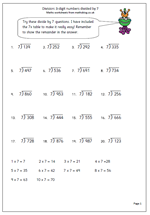Here is another page for practising the short division method. When I was young I was taught the short method before the long method and by repeated practice learned the method, although probably not knowing why it works. Today the long method is usually introduced first as a means of showing exactly how it works, usually with easier numbers. The key to both methods is, of course, a good knowledge of tables, although on this page I have written out the 7 times table to help.

For example: 7)627

‘How many sevens in 62?’

‘7 times 8 is 56, 7 times 9 is 63.’

‘7 goes into 62, 8 times with 6 left over.’

Write the 8 in the answer above the 2.

Write the remainder 6 beside the 7 units, making 67.

‘How many sevens in 67?’

‘9 times 6 is 63.’

‘7 goes into 67, 9 times with 4 left over.’

Write the 9 in the answer in the units and write rem 4 next to it.

Division 3-digit numbers by 7

## Resource of the Week: half way between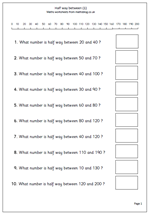This week I am highlighting a page which, on the face of it this looks quite a simple task, but many children (and adults) find very tricky. The page is on how to work out what half way between two numbers is. Often there is a lot of ‘trial and improvement’ going on in people’s heads as they guess their way towards finding half way.

Two methods make the task fairly straightforward.

For method one a number line is very useful to start with. Put a finger of the left hand on the first (lower) number shown on the number line and a finger of the right hand on the second number. Then move the left hand finger one place to the right and the right hand finger one place to the left. Repeat this until the two fingers meet – that is your half way number.

The second way of finding half way involves two steps:

step 1: add the two numbers.

This is the better method as it works for all numbers, not just those shown on the number line.

This page can be found in our Year 3, Counting and Number section

Half way between_(1)

## Short multiplication by 6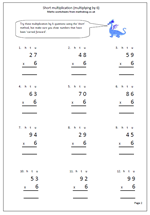Here is the latest worksheet which looks at the short multiplication method; this time multiplying 2-digit numbers by 6. It is called the short method because it eliminates the process of putting parts of the answer in two or three columns below, as in the ‘long’ method. The stages are the same as before:

1. writing the question out correctly so that it is in vertical format. it is a good idea to write a small h, t and u above to show the hundreds, tens and units columns.

2. Multiplying the units and carrying any of the tens across, below the answer line.

3. multiplying the tens and putting any hundreds into the answer.

More clarification of this method is shown on the worksheet.

The 6 times table is one of the harder tables to learn, but knowing it off by heart makes this type of multiplication so much easier.

Short multiplication by 6

## Counting games for Year 1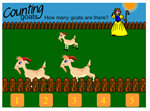Whilst most of the mathsblog site gives away free worksheets I do have a small selection of fun maths games for reception/Year 1 children. Counting is a major part of maths iat this age and by the end of reception should be able to say and use number names up to 10. A good example of this would be to join in nursery rhymes or songs such as

“One, two three, four, five. Once I caught a fish alive.”

Children should also  be counting up in ones, up to 10 objects. This would be in a practical sense of counting a number of objects that they can touch (pieces of a jigsaw, coins, hats etc).  Later they can count things they can see, but not touch (panes in a window, cows in a field etc ).They can then begin to count down from a small number e.g. 5,4,3,2,1,0.

We have a great set of fun maths games on counting for Reception/Early Years and one of my particular favourites is the Counting Goats game. This is really good practice at counting up to 5. Young children can not get too much practice with this both in the home and on the computer. They may well use their fingers to help and count out loud, but after a time they will begin to count in their heads. Adults can often glimpse at a picture to see how many there are, almost without counting – don’t expect this of 5 a year old! Don’t forget to click on the banjo playing goat at the end for a happy tune!

We have a great set of counting games, so why not have a go today?

Go to our Counting games

If you like these games why not have a free trial of urbrainy.com, which has many, many more?

## Useful resources: 0 to 99 number grid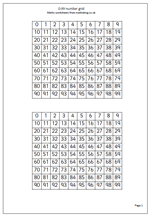I recently published a number grid (or number square) from 1 to 100 and today I am publishing a similar page, but for numbers from 0 to 99.

Number grids can be used in a wide variety of ways, and many patterns can be found. it shows clearly the pattwern of odd and even numbers as well as the patterns created by multiplying. For example, colour squares green that are multiples of 2; colour squares red that are multiples of 3 and colour squares green which are multiples of 4. Which squarea are coloured more than once? Why? etc.

0-99 number grid